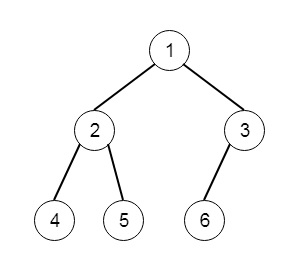# Count Complete Tree Nodes in C++

Suppose we have a complete binary tree, we have to count the number of nodes. So if the tree is like −So the output will be 6.

To solve this, we will follow these steps

• This will use the recursive approach. This method, countNodes() is taking the root as argument.
• hr := 0 and hl := 0
• create two nodes l and r as root
• while l is not empty
• increase hl by 1
• l := left of l
• while r is not empty
• r := right of r
• increase hr by 1
• if hl = hr, then return (2 ^ hl) – 1
• return 1 + countNodes(left of root) + countNodes(right of root)

Let us see the following implementation to get better understanding −

## Example

Live Demo

#include <bits/stdc++.h>
using namespace std;
class TreeNode{
public:
int val;
TreeNode *left, *right;
TreeNode(int data){
val = data;
left = right = NULL;
}
};
void insert(TreeNode **root, int val){
queue<TreeNode*> q;
q.push(*root);
while(q.size()){
TreeNode *temp = q.front();
q.pop();
if(!temp->left){
if(val != NULL)
temp->left = new TreeNode(val);
else
temp->left = new TreeNode(0);
return;
}else{
q.push(temp->left);
}
if(!temp->right){
if(val != NULL)
temp->right = new TreeNode(val);
else
temp->right = new TreeNode(0);
return;
} else {
q.push(temp->right);
}
}
}
TreeNode *make_tree(vector<int> v){
TreeNode *root = new TreeNode(v);
for(int i = 1; i<v.size(); i++){
insert(&root, v[i]);
}
return root;
}
class Solution {
public:
int fastPow(int base, int power){
int res = 1;
while(power > 0){
if(power & 1) res *= base;
base *= base;
power >>= 1;
}
return res;
}
int countNodes(TreeNode* root) {
int hr = 0;
int hl = 0;
TreeNode* l = root;
TreeNode* r = root;
while(l){
hl++;
l = l->left;
}
while(r){
r = r->right;
hr++;
}
if(hl == hr) return fastPow(2, hl) - 1;
return 1 + countNodes(root->left) + countNodes(root->right);
}
};
main(){
Solution ob;
vector<int> v = {1,2,3,4,5,6,7,8,9,10};
TreeNode *node = make_tree(v);
cout << (ob.countNodes(node));
}

## Input

[1,2,3,4,5,6,7,8,9,10]

## Output

10